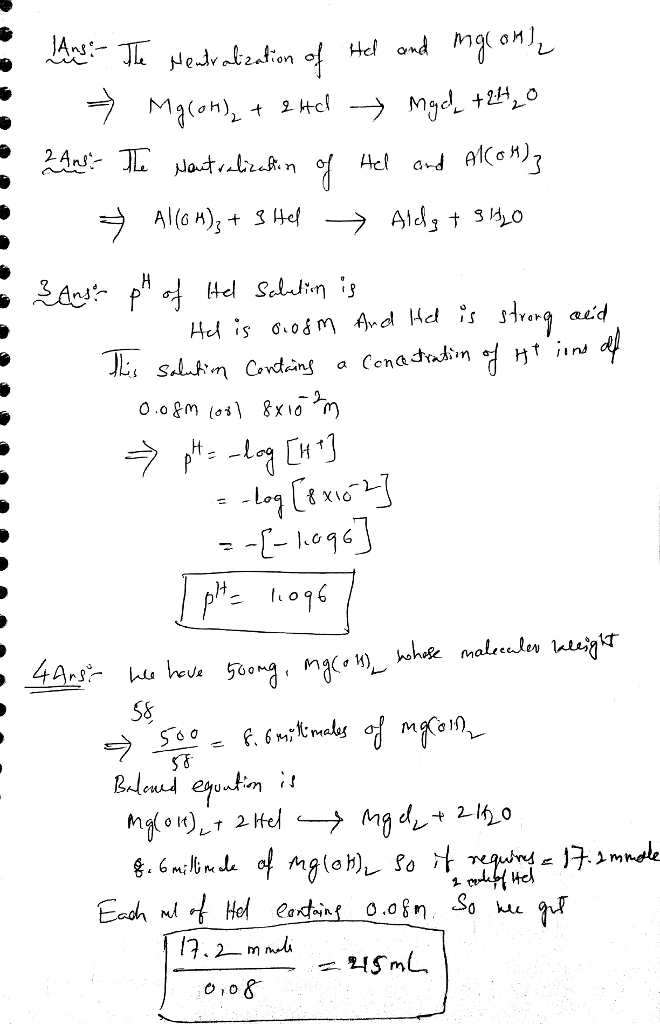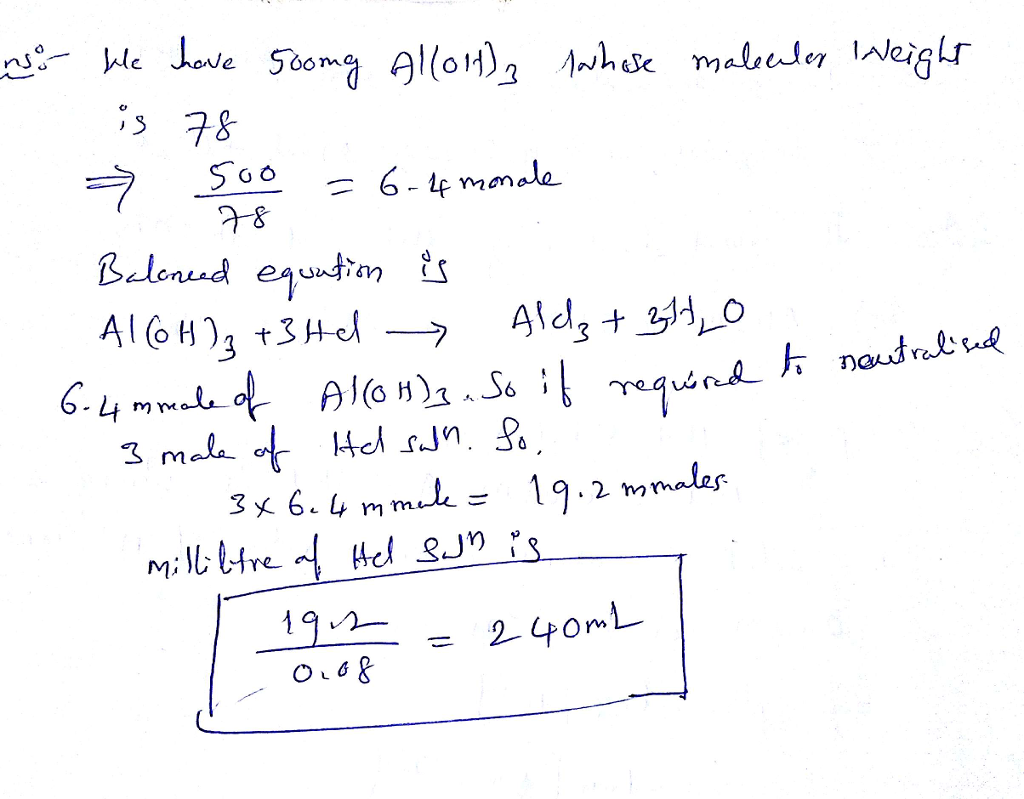# Question & Answer: In a teaspoon (5.0 ml) of a liquid antacid there are 500. mg of Mg(OH)2 and 500. mg of…..

In a teaspoon (5.0 ml) of a liquid antacid there are 500. mg of Mg(OH)2 and 500. mg of Al(OH)3. A 0.080 M HCl solution. which is similar to stomach acid, is used to neutralize 10.0 ml of liquid antacid.
1. Write the equation for the neutralization of HCl and Mg(OH)2
2. Write the equation for the neutralization of HCl and Al(OH)3
3. what is the PH of the HCl solution?
4. how many milliliters of the HCl solution are needed to neutralize the Mg(OH)2?
5. How many milliliters of the HCl solution are needed to neutralize the Al(OH)3 solution?